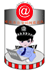波形护栏板被撞后怎么修复

1.首先我们通过观察，卸掉受损的波形护栏板，然后检查附近的波形护栏板与立柱是否受到了损害，有时我们无法用肉眼检查出来，这时我们就需要经过专业工具测试一下，周边的护栏板是否能达到防护的效果。

2.卸掉受损的波形护栏板后，我们需要安装新的波形护栏板，安装的时候我们需要做到完美的拼接，保证防撞等级系数，这样才能起到防护的效果。

3.如果立柱受损后我们需要更换立柱，埋设立柱后应尽量把土压实，并保证不低于之前的路面。

4.*需要将立柱的防雨帽盖上。

(一)二波板一、九孔普板以一段100米为例列举所需的材料。

1、护栏板(85*310*4.0*4320)：总长度4=100÷4=25片

2、立柱：总长度÷4+1=26根(每一段加一根，若一百米为二段，则加二，以此类推)

3、柱帽：柱子数量*1=26个

4、防阻块或托架：柱子数量*1=26个

5、横梁垫片(常用44*76)：柱子量*1=26块

6、连接螺栓M16*170：柱子数量*1=26套

7、连接螺栓M16*45：柱子数量*1=26套

8、拼接螺栓M16*35：护栏板数量*8=25*8=200套

9、端头(常用R160)：段数*2=1*2=2个

1、护栏板(85*310*4.0*4320):总长度÷4=100÷4=25片

2、立柱：总长度÷4+1=26根(每一段加一根，若一百米为二段，则加二，以此类推)

3、柱帽：柱子数量*1=26个

4、防阻块或托架：柱子数量*1=26个

5、横梁垫片(常用44*76)：柱子数量*2=52块

6、连接螺栓M16*170：柱子数量*1=26套

7、连接螺栓M16*45：柱子数量*2=52套

8、拼接螺栓M16*35：护栏板数量*8=25*8=200套

9、端头(常用R160)：段数*2=1*2=2个四、十孔加强板(立柱2米一根)